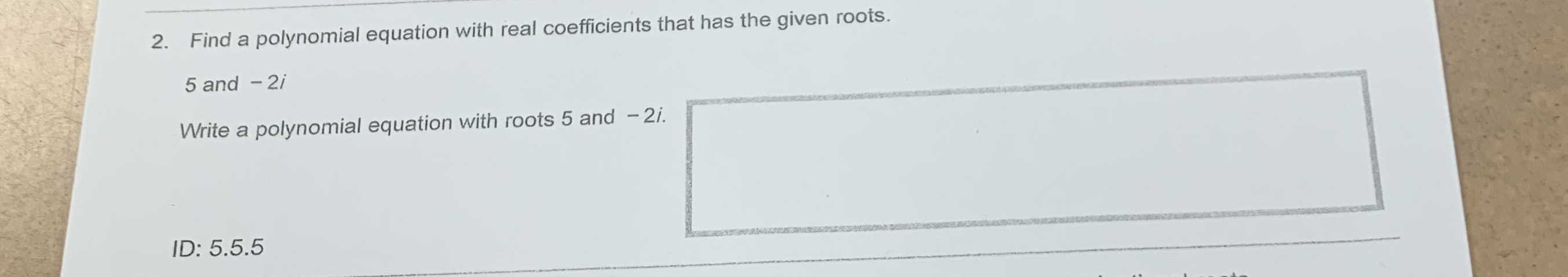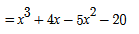### ¿Todavía tienes preguntas de matemáticas?

Pregunte a nuestros tutores expertos
Algebra
Pregunta2. Find a polynomial equation with real coefficients that has the given roots.

$$5$$ and $$- 2 i$$

Write a polynomial equation with roots $$5$$ and $$- 2 i .$$